﻿ Study of the Power Consumption of a Digital-Front-End Using Random Sampling

### Study of the Power Consumption of a Digital-Front-End Using Random Sampling

Deng Xiaoyu, M. Diop, J.F. DiourisOPEN ACCESSPEER-REVIEWED

## Study of the Power Consumption of a Digital-Front-End Using Random Sampling

Deng Xiaoyu1, M. Diop2,, J.F. Diouris1

1Ecole polytechnique de l’Université de Nantes, Rue C. Pauc, La Chantrerie, Nantes, France

2Ecole Supérieure Polytechnique, UCAD, Dakar-Fann, Sénégal

### Abstract

Recently, irregular sampling techniques have been proposed for the design of digital front-end of a radio receiver. This front-end consist in the interface between the analog front-end and the baseband processing. The advantage of these techniques is the simplification of the sampling frequency conversion and the channel selection. The objective of the proposed work is to study if a gain in power consumption is also obtained. In this paper, the major research is the digital-front-end power consumption by using random sampling. Firstly, we introduce the methods of random sampling JRS (Jitter random sampling) and ARS (Additive random sampling). Then we use these methods to generate the random clock, select the hardware as mixed platform with ADC and FPGA and implement different solutions. At last, we measure the power consumption of different solutions and make a comparison.

### At a glance: Figures

• Xiaoyu, Deng, M. Diop, and J.F. Diouris. "Study of the Power Consumption of a Digital-Front-End Using Random Sampling." International Transaction of Electrical and Computer Engineers System 2.2 (2014): 73-80.
• Xiaoyu, D. , Diop, M. , & Diouris, J. (2014). Study of the Power Consumption of a Digital-Front-End Using Random Sampling. International Transaction of Electrical and Computer Engineers System, 2(2), 73-80.
• Xiaoyu, Deng, M. Diop, and J.F. Diouris. "Study of the Power Consumption of a Digital-Front-End Using Random Sampling." International Transaction of Electrical and Computer Engineers System 2, no. 2 (2014): 73-80.

 Import into BibTeX Import into EndNote Import into RefMan Import into RefWorks

### 1. Introduction

Before digital signal processing, the signal must be presented in the appropriate digital format. Therefore the original analog signal, before processing, has to be converted into a digital one, i.e. it has to be digitized. Once a signal is digitized, the features of the obtained digital signal are fixed and nothing can be done to change them. In an ideal world, these features would exclusively depend and actually would copy the features of the original analog signal. The reality is different. The two basic operations of any analog to digital conversion, both sampling and quantization, impact the characteristics of the digital signal substantially. The characteristics of the analog signal at the ADC input and of the digital signal at its output are just similar rather than identical. How large and significant the difference between them depends on the digitizing methods and their implementations.

Information carried by an analog signal can be represented in a digital form by a sequence of instantaneous values measured at discrete time instants. These signal readings are usually considered as signal sample values and the process of taking them is referred as sampling. The instants at which the samples are obtained form a stream of events, which can be demonstrated graphically as a sampling point process. Characteristic features of the sampled signals to a large extent depend on the patterns of the point processes generated and used for sampling. When sampling is mentioned in the technique of DSP, usually it is assumed that it is deterministic and uniform (equidistant). The model of sampling according to which signal samples are separated by time intervals with a constant and known duration is the most popular. This is readily comprehensible because such a sampling approach appears to be the most natural and obvious. It also has a number of attractive advantages.

However, as was established relatively long ago, the application of periodic sampling alone is not sufficient. The periodic sampling model is not applicable when fluctuations in sampling instants cannot be ignored or when signal samples can be obtained only at non-uniform or even random time intervals. Studies have indicated that randomness in sampling is not always harmful, random irregularities in sampling sometimes can even be beneficial . These irregularities, if properly introduced, provide, for instance, such a useful effect as the suppression of aliasing. And such sampling itself usually is considered as non-uniform. Depending of the required performance specification of the signal processing system to be developed and given both in functional and performance quality terms, sampling can be adapted by taking the following decision: the choice of either periodic or non-uniform sampling.

While periodic sampling is preferable, in high frequency signal processing cases, the required periodic sampling rate might be too high. Then usage of non-uniform sampling might be better. However special and more complicated signal processing algorithms have to be used in that case. Therefore adapting the sampling operation to the specific signal processing conditions comes down to a trade-off between the complications caused either by high sampling rate or more complex algorithms.

Uniform sampling is preferable whenever the spectrum of the signal can be restricted as required by the Sampling Theorem. Firstly, periodic sampling is the simplest method of performing this procedure and is easy to implement. Secondly, periodic sequences of signal samples are well suited to digital processing. Note only that, many highly efficient fast algorithms are applicable.

Random sampling may prove to be more profitable when it is undesirable or even impossible to pre-filter signals before their analog digital conversion, when the signal to be processed contains components at frequencies exceeding half of the sampling rate. However the essence of randomized sampling is taking the signal sample values at unknown random time instants. Therefore application of randomized sampling is limited to the relatively cases where the information about the exact sampling instants is not relevant.

Pseudorandom sampling is the most often used non-uniform sampling based anti-aliasing technique. The indications for its usage are the same as for the randomized sampling except that the sampling instants in this case are known with high precision.

The significance of the sampling operation is determined by the fact that many essential digital signal characteristics, impacting the whole signal processing process substantially depend on the methods and techniques used to perform it. In the traditional DSP case, the only way to reduce this often undesirable dependence is increasing the sampling frequency. However, the possibilities then are poor and limited. In addition, this approach in many cases produces an increased number of bits requiring more complicated hardware for the subsequent processing of the obtained in these way digital signals. Deliberate introduction, when necessary, of an element of randomness into the sampling operation helps a lot in obtaining the flexibility essential for adaptation of the analog-digital conversion to the conditions of the given specified signal processing task. Therefore the application of non-uniform sampling is more flexible then use of traditional uniform sampling.

In this paper, we propose to use the random sampling method implemented by original design of a Pseudorandom Signal Sampler circuit for controlling ADC to relax constraints of receiver circuits supporting multiband signal processing. This new idea of using non-uniform sampling technique for multiband signals sampling allows the main advantage of suppressing spectral aliases at integer multiples of sampling frequency produced by conventional uniform sampling technique. We expect this reduces the constraints on the anti-aliasing filter, relaxes the automatic gain control dynamic range, and decreases the ADC dynamic power consumption.

Non-uniform sampling theory and techniques are presented in various publications [1, 2, 3] and used for some applications such as duty cycle measurement and spectrum analysis. For practical implementation of non-uniform sampling, some non-uniform signal sampler solutions are proposed in the literature [4, 5, 6, 7].

The rest of the paper is organized as follows. In section two the theory of random sampling is presented. Three methods of generation of the non-uniform clock and implementation with VHDL on FPGA are detailed in the section three. An analysis of power consumption is presented in section four and comparison is done under different frequencies and using regular and irregular sampling. Finally, a conclusion is given with some directions for future works.

### 2. Theory of Random Sampling

The properties of random sampled signals are mainly defined by the mode of generation of point streams used for the implementation of sampling, that is, the selection of points in time for signal readout. Although there are a relatively large variety of known non-equidistant spaced point processes, only a few of them actually have the characteristics required for high performance sampling. The available expertise in application of various random point streams suggests that the most advisable technique for producing sampling instants is based on additive random point process. It is really well suited for the purposes of deliberate sampling randomization. This point process has such remarkable properties and is so flexible that it suits random sampling applications very well. Specifically, sampling carried out in this way ensures that all parts of any input signal are sampled with equal and constant probability. Therefore such sampling is signal-independent. And it provides for unbiased (no systematic errors) estimation of signal parameters, including spectral parameters. However, there are several other point processes which should also be considered because they are connected to relatively frequently observed and important effects such as fluctuation of uniform sampling instants or random selection of uniformly spaced samples.

2.1. Jitter Random Sampling

Fluctuation of sampling instants is a fairly common occurrence . It can even be said that it is always present. In the case of uniform sampling with jitter, signal samples {} are taken at time moments=kT+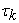, T>0, where T is a period of uniform sampling, but {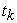} is a family of identically distributed independent random variables with zero mean. This sampling scheme is illustrated in Figure 1. It shows the probability density functions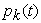of time intervals (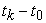). As can be seen from Figure 1(d), this particular function has multiple peaks. Note that as t increases the peaks shown do not decrease.

To understand the meaning of the function, imagine that a narrow time window t is moved along the t-axis. Under the condition t 0, the functionat any arbitrary time instant is equal to the probability that one of the sampling points will fall within this window. Therefore if a signal is sampled at the instants which are determined by the statistical relationship illustrated by Figure 1 (d) some parts of the signal will be sampled with a higher probability than others. This is obviously undesirable, as it will lead to the signal processing errors. There is an exception. If the time intervals () are distributed uniformly in the intervals (kT ± 0.5T) respectively, then the resulting sampling point density function is constant. But in fact this method of generating non-uniform sampling point stream has a number of substantial disadvantages, which prevent its wide application:

•  The random variables {} should be distributed strictly uniformly within the given intervals

•  Time intervals between any two sampling instants may be very short.

Figure 1. Probability density functions characterizing periodic sampling with jitter. a), b), c) probability density functions of a sum of 1, 2 and 3 time intervals, respectively; d) resulting sampling point density function

In the case of additive random sampling, signal samples are taken at time moments, where {} is a family of identically distributed independent positive random quantities . Such a non-uniform point stream can be easy implemented to suppress the overlapping effect. The degree of randomization can be varied through appropriate selection of only one parameter, where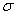and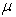are the mean value and standard deviation of the intervals between sampling points, respectively. Obviously the mean sampling rate is equal to. The additive random sampling scheme itself is illustrated by Figure 2.

Probability density function of time interval: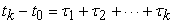in this case can be calculated as, where the asterisk * denotes the convolution operation, and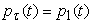is a density function of {} distribution. As the random variablerepresents the final result of a linear sum of statistically independent constituent variables, then whatever probability density function these variables may have, the probability density of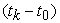will approach the normal form as k approaches infinity. As can be seen from Figure 2, the sampling point density function in the case of additive non-uniform sampling with t increasing will always tend to become flat. The value of this constant level is equal to 1/μ. When non-uniform sampling is selected for application in a given case, an appropriate sampling rate has to be set up. The criteria for the choice of an appropriate sampling rate, in the case of non-uniform sampling, completely differ from those commonly used for periodic sampling. The spectral component of the signal with highest frequency to be non-uniformly sampled and processed actually is not a criterion then. The mean sampling rate is calculated by evaluating the number of signal samples needed and the longest time interval during which those samples have to be acquired for one signal realization.While the minimum of the signal samples to be taken at periodic sampling actually is equal to the required number of them in the case of non-uniform sampling, excessive samples often are taken at periodic sampling just to avoid aliasing .

Figure 2. Probability density functions characterizing additive random sampling. a), b), c) probability density functions of a sum of 1, 2 and 3 time intervals, respectively; d) resulting sampling point density function
2.3. Multiband Signal Reconstruction Based on SVD

The multiband signal x= x(t) has a Fourier transform vanishing outside of measure B . Using ARS as the sampling method, write T for the observation interval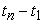. A reconstruction of the following form is:(1)

In which the () lie in the B bandwidth and with in each subinterval, are equally spaced. Theis found by minimizing the square-error(2)

The optimum is obtain from the following formulary:(3)is the conjugate matrix of A.

With the matrix calculation, we can obtain: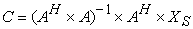(4)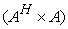is a singular matrix, so we should use a suitable algorithm to solve C.

SVD (singular value decomposition) method is a proper way to resolve the problem. SVD algorithm decomposes A into three matrices: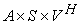(5)

U and V are matrices with dimension N and M, in addition,(6)

S is a diagonal matrix: S=diag(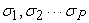), and p=min(M,N),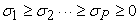.

Suppose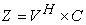and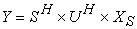, the Eq. (3) is equivalent to: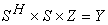(7)

then we can obtain: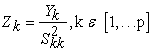(8)

Finally, we can obtain the coefficients: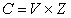(9)

In , the following empirical results were obtained:

• Good reconstruction was obtained when the design matrix is of full column rank, that is, the columns are independent, and rank (A)/m = 1. A necessary condition is that the sampling rate exceed the Nyquist-Landau rate (or else n < m and the matrix cannot be of full column rank; the rank of a matrix cannot exceed either of its dimensions).

• Regular sampling, sampling in allowed region. The functions are independent with regard to the sampling set, and rank (A)/m =1.

• Regular sampling, sampling rate in disallowed region but above the Nyquist-Landau rate. The functions become folded on top of each other in frequency space, and the columns of A become dependent; therefore, rank (A) falls by an amount proportional to the degree of overlap. However, rank (A)/m> (1/2) because the maximum degree of overlap is seen when one band sits exactly on top of the other, and then, only half the columns become redundant.

• Random sampling. When the average sampling rate was a little over the Nyquist-Landau rate (q < 1; we found q = 0.9 to give acceptable results), the design matrix was of full column rank, and good reconstruction was obtained. However, we did not investigate the effect of having large gaps in the sampling; one would expect these to significantly reduce the quality of reconstruction.

We consider the reconstruction of a multiband of five QPSK modulated carrier signals, show in the Figure 3, each at a symbol rate Rsym =4 sym/s and with a raised cosine filter excess bandwidth factor of 0.5. Each carrier is separated by 8 Hz; therefore, the group band occupies a theoretical bandwidth of 38 Hz. We construct the original signal using a fine regular sampling grid () at 1000 Hz. A typical realization of the signal and its power spectral density (PSD) is shown in Figure 4 when the center frequency is 175Hz.

To obtain the sampled signals, we decimate the original sample set in two ways: regularly and irregularly. In the first case, the sampling rate is 100 Hz; in the second, the average sampling rate is 100 Hz. So we sample the QPSK signal by using regular, ARS and JRS and reconstruct the signal based on SVD.

Figure 5 illustrate the signal sampled by ARS and reconstruct with SVD.

In Figure 6, it show that signal sampled by JRS and reconstruct with SVD.

And in Figure 7, it demonstrates the regular sampling and reconstructs the signal using SVD.

We also calculate the average SNR and error of each way:

SNR (ARS) = 58.657809, E (ARS) =0.0109

SNR (JRS) =59.662221, E (JRS) =0.0076

SNR (unif) =67.479956, E (unif) =0.0066

With these result, we can consider that the randomized sampling scheme can be used for the multiband signal without the restrictions on sampling rate.

### 3. Generation of Random Clock and Implementation of FPGA

Based on the previously chapter, we have two ways to generate the random sampling. In the following parts, we introduce three methods to generate the non-uniform clock, and implement with VHDL on FPGA.

3.1. Pseudorandom Clock Generation
3.1.1. Non-uniform Processing Formulation

In , non-uniform sampling process, used in this work, converts a continuous analog band pass signal x(t) into its discrete representation xs(t) as describe in eq.(10):(10)

The sampling instant sequence {} is defined as {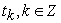}{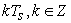} withthe mean of the sampling period. This random sequence can be defined by either jitter random sampling (JRS) , or additive random sampling (ARS) . To obtainin JRS scheme, we add a random timeto deterministic instants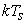. However, to obtainin ARS scheme, we addto the previous sampling instant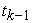. If irregularities are appropriately chosen, they could provide the aliasing suppression. Alias-free processing is met when the sampling point density function assumes a constant value equal to the mean sampling frequency fs given by equation (11):(11)

whereis the probability density function of the random sampling point. This stationary condition is accomplished in case of ARS scheme, and in case of JRS scheme only for uniform probability density over [].

Nevertheless, random sampling is not convenient to generate and precisely recover uniform sampling instants. In most non-uniform sampler implementations, the sampling instants are, either randomly or pseudo randomly, analogically generated. Then, the sampling generated instants are digitized before being used in digital recovering process . In , Wojtiuk proposed the time quantized random sampling scheme. Each random time, is quantized to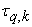and represented by Eq. (12) according to Eq. (13):(12)(13)

Where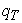is the quantization time factor and n a positive integer number. The TQ-RS in case of JRS scheme for= 4 is illustrated in Figure 8 .

3.1.2. Circuit of Pseudorandom Signal Sampler

To sample non-uniformly, we define several sampling phases instead of conventional uniform sampling. The solutions based on phases, noted {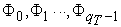} and it present the required phases for TQ-RS for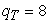in Figure 9. The delay between the two successive clock phases is the minimum spacing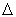defined in Eq. (12), and the phases duty cycles are equal.

We propose a design to generate the random clock using these different phases but the same frequency clocks. Figure 10 illustrates the block diagram of the circuit .

Counter and combiner

To overcome overlap, it is better to use gray counter outputs combined to deliver the needed phase for the non-uniform sampling. The sampling time is the rise edge of the available phases. The counter is controlled by CLK and is based on a shift register withflip-flops. In this case, the resulting generated phases for non-uniform sampling are presented in Figure 9.

Linear Feedback Shift Register (LFSR)

Phases generated, from the counter and combiner, are selected according to a random number. Linear feedback shift registers make extremely good pseudorandom pattern generators. When the outputs of the flip-flops are loaded with a seed value (anything except all 0, which would cause the LFSR to produce all 0 patterns) and when the LFSR input a clock, it will generate a pseudorandom pattern of 1and 0. A LFSR with n length characteristic polynomial G[x] generates a binary pseudorandom sequence each period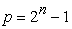when initial state is non zero. LFSR synthesis requires at least n flip-flops. Here we propose to generate pseudorandom numbers using an LFSR composed offlip-flops at CLK/rate. In our previous example, we need an LFSR as represented in Figure 11. The characteristic polynomial is given by Eq. (14)(14)

Selective combiner

Having the different sampling phases and the pseudorandom number, we have to introduce a selective combiner to select the phase according to the delay iΔ where i is the pseudorandom number generated by the LFSR being in the set {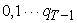}. The selective combiner is a multiplexerinputs to one output controlled by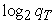signals

General results

In Figure 12, phases generated by the counter and combiner (), pseudorandom numbers "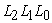" and the result pseudorandom clock CLK_NUS are given.

3.2. Random Clock Generation

Previously in the chapter 3，we use pseudorandom number to generate the non-uniform clock, the algorithm of the method is approximate JRS. In the following part, we propose to generate another random clock, it is approximate ARS.

Figure 13 gives the architecture of the circuit.

In this case, the component of Random clock generator contains a register, in Figure 14, which have N bit, and from the N-1 to N-M-1.

Bits are ‘, the others are ‘. From this register, we can define the average sampling frequency: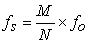(15)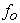is the frequency of FPGA original clock.

With the LFSR, we propose to use the longer length LFSR. Because with this we can generate the long periodic number which can improve the sampling to the full extent of randomization. In this design, when the LFSR generate a number, meanwhile it will select the correspond position in the register, and if the position is ‘, the clk_out will output ‘1’.

For example, if N=32 and M=7, the LFSR can generate 5 bits pseudorandom numbers, with the program; in Figure 15, there is the simulation of the application.

Furthermore, if we want to obtain more precise result, we can modify N and M, for instance, N = 512 and M = 10, LFSR can generate 9 bits pseudorandom numbers, according to Figure 16, the simulation show that pseudorandom sampling in long period is better than in short one, it is more close to the real random sampling.

### 4. Comparison of the Power Consumption

Based on the generation of random clock, we can make a measurement of power consumption with ADC and FPGA platform, and comparison the power consumption under different clock frequencies, also compare the regular sampling and irregular power consumption.

4.1. Results of Using Pseudorandom Clock

The input signal to ADC equal to 750 KHz, amplitude is 2.6V. We measure the voltages and calculate the currents with sampling frequency equal to 1.56MHz, 3.12MHz and 6.25MHz. In Figure 17 - Figure 18, it demonstrates the results both uniform and non-uniform.

4.2. Results of Using Approximately Random Clock

The input signal of ADC equal to 500 kHz, amplitude is 2.6V. We measure the voltages and calculate the currents with a sequence of sampling frequency. Figure 19 - Figure 20 illustrate the results both uniform and non-uniform.

### 5. Conclusion

According to these result above, we can observe in Figure 18 - Figure 20 that the current increases when the sampling frequency increases. While compare the currents between the uniform and non-uniform, the former is larger than the latter one.

Compare the results which are deduced from the two kind of non-uniform clocks, when we use the same sampling frequency, the current is not the same. Aim to prove the second random clock is more precise than the first one, with the second clock used in &3.2, we propose to M=1&N=8 and M=16&N=128; with these two groups, the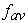both equals to 6.25MHz. Implemented on the ADC, the first group M&N obtains the result as same as using the first method (&), while with the second group M&N, the result I measured is lower than the result of first group. Obviously we can consider that the second method is more precise than the first one.

In conclusion, with nonuniform clock, the current is lower than using the uniform clock, we can consider that random sampling can decrease the ADC dynamic power consumption.

### Future Work

In this case, if we can verify the reconstruction of the signal with the random sampling, it will be more compellent. So I propose to obtain the samples and sampled time form the FPGA card and demonstrate in the oscillograph, to be certain that using random sampling can decrease the power consumption of ADC and also reconstruct the signal completely. After these work, we can continue to measure the power consumption on FPGA and make comparison among the different situations.

### References

  J.J.Wojtiuk, Randomized Sampling for Radio Design, "Ph.D.Thesis", University of South Australia, School of Electrical and Information Engineering,2000.In article  Jeffrey J. Wojtiuk and Richard J. Martin,"Random Sampling Enables Flexible Design for Multiband Carrier Signals,"IEEE TRANSACTIONS ON SIGNAL PROCESSING, VOL. 49, NO. 10, OCTOBER 2001.In article  R.J. Martin and D.A. Castelow, "Reconstruction of multiband signals using irregular sampling", GEC J. Tech., vol. 14, pp. 180–185, 1997.In article  Manel BEN-ROMDHANE, Chiheb REBAI, Adel GHAZEL,"Pseudorandom Clock Signal Generation for Data Conversion in a Multistandard Receiver", 2008 International Conference on Design & Technology of Integrated Systems in Nanoscale Era.In article  Chiheb Rebai, Manel Ben-Romdhane&al, "Pseudorandom signal sampler for relaxed design of multistandard radio receiver," Microelectronics Journal 40 (2009)", pp.991-999.In article CrossRef  N.Michael, S.Shah, J.Das, M.M.Sandeep, C.Vijaykumar, "Nonuniform digitizer foralias-free sampling of wide band analog signals",in IEEE Region 10 Conference,TENCON2007,October–November 2007, pp. 1-4.In article  Haïfa FARES, Manel BEN-ROMDHANE, Chiheb REBAI,"Non Uniform Sampled Signal Reconstruction for Software Defined Radio Applications", 2008 International Conference on Signals, Circuits and Systems.In article